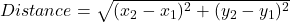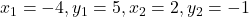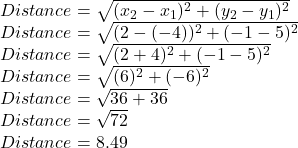Question

What is the length of segment TR with T(-4,5) and R(2, -1)​

1.The length of segment TR with T(-4,5) and R(2, -1)​ is 8.49

Step-by-step explanation:

We need to find the length of segment TR with T(-4,5) and R(2, -1)​

The length of segment can be found using distance formula.

The distance formula is:Putting values and finding length

We have:We found Distance = 8.49

So, The length of segment TR with T(-4,5) and R(2, -1)​ is 8.49.